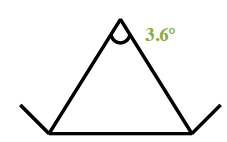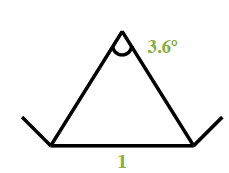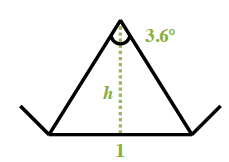### Home > GC > Chapter 10 > Lesson 10.1.2 > Problem10-18

10-18.

Find the area of a regular polygon with $100$ sides and with a perimeter of $100$ units.

A triangle, with top vertex labeled, 3.6 degrees. Find the measure of a central angle.

$\text{Central angle}=\frac{360^{\large\circ}}{100}=3.6^{\large\circ}$

Determine the length of the sides, which will be the length of the base of the triangles.

If $P=100$, $\frac{100 \text{ units}}{100 \text{ sides}}=1$ unit per side Label, of 1, added to bottom side of triangle.

Added: A dashed line, labeled h, from the top vertex, perpendicular to the bottom side. Use the tan trig ratio to determine the height of the triangle. Use this and the length of the base to find the area of the triangle.

$\frac{3.6^{\large\circ}}{2}=1.8^{\large\circ}$$\tan1.8^{\large\circ} =\frac{\text{opp}}{\text{adj}}=\frac{0.5}{h}$$h=\frac{0.5}{\text{tan}1.8^{\large\circ}}\approx 15.9103$

$A=\frac{1}{2}(1)(15.9103)\approx 7.9551 \text{ units}^{2}$ for each triangle.

Use this area to determine the area of the entire polygon.

$\text{Area}≈ (100)(7.9551)$Area $≈ 795.51\text{ square units}$